﻿ Gravitational Mass Defect

### Gravitational Mass Defect

F. F. MendeOPEN ACCESSPEER-REVIEWED

## Gravitational Mass Defect

B.I. Verkin Institute for Low Temperature Physics and Engineering NAS, Ukraine, Lenin Ave., Kharkov, Ukraine

### Abstract

Explosive energy in the nucleus of the galaxy NGC 3034 composed 9 • 1048 J. In this case of its nucleus of galaxy was rejected the mass equal to 5,9 • 107 of the masses of the sun. At present are not known the physical mechanisms, which can explain such immense explosions. In the article is examined the new physical phenomenon, gravitational mass defect, which can explain the phenomenon indicated. This phenomenon leads to the fact that the summary mass of bodies before and after the collisions differ. Indicated we will call mass defect gravitational mass defect and it can serve as a reason for explosions in the nuclei of galaxies.

### Cite this article:

• Mende, F. F.. "Gravitational Mass Defect." International Journal of Physics 3.1 (2015): 29-31.
• Mende, F. F. (2015). Gravitational Mass Defect. International Journal of Physics, 3(1), 29-31.
• Mende, F. F.. "Gravitational Mass Defect." International Journal of Physics 3, no. 1 (2015): 29-31.

 Import into BibTeX Import into EndNote Import into RefMan Import into RefWorks

### 1. Introduction

With the supernova explosions stars their luster increases by tens of stellar magnitude in the course several days. In the maximum of luster the supernova is compared in the brightness with the entire galaxy, in which it exploded even to exceed it. So the luminosity supernova SN1972E almost 13 times exceeded the integral luminosity of the galaxy NGC5253, in which occurred explosion . Therefore the explosions of supernovas it is possible to record with the very great distances up to 1000 Mpc, and even further . During the supernova explosion is separated energy on the order of 1043 - 1044 J . Of the final theory supernova thus far there does not exist; therefore it is not clear to the end, what energy sources lead to the such immense explosions.

In 1963 american astronomers to Linda and Sendidzh published the results of investigating the galaxy NGC 3034. A study of the photographs of galaxy showed the presence of the dense system of dark channels and bright fibers of diffuse material, connected with the nucleus, which testify with their form about the energetic motion, that are stretched up to the distance to 3 kps on both sides from the nucleus. Spectrograms showed that diffuse material gives emissive lines and, therefore, some mechanism put gas into the excited state. Emissive lines reveal expansion. Measurement showed that gas moves with a speed of about 1000 km/s, forming fibers. Since fibers break themselves at a distance by 3 kpc from the nucleus (gas it had time to reach this place), then all enumerated phenomena made it possible to arrive at the conclusion that in the nucleus NGC 3034 about one-and-a-half million years ago occurred the immense explosion, which caused ejection with a speed of about 1000 km/s of the enormous masses of diffuse material. The energy, which was released during explosion, was spent to that in order to set into the rapid motion diffuse material, and also that to convert its atoms to the ionized state.

In the observed intensity of emission in the line Ha it was possible to estimate the density of the rejected diffuse material and, consequently, also its overall mass, which proved to be equal to 5,9 • 107 of the masses of the sun. This makes it possible to value general kinetic energy of the moving diffuse material, which composed 2,4, • 1048 J. If we estimate total power, entire emission and to assume that for all one-and-a-half million years from the beginning of explosion the radiated power was constant, then the estimation, the explosive energy, spent for the emission to the present moment, were equal to 9 • 1048 J.

Therefore it is possible to give the estimation of general explosive energy in the nucleus NGC 3034. This energy composed 1049 J, T. e. in millions of times it is more than the energy, isolated with the supernova explosion star. Previously supernova explosions were considered as the most immense catastrophes in the universe, but explosive energy in the galaxy NGC 3034 is into millions of times more than the explosions of supernovas .

At present are not known the physical mechanisms, which lead to the such immense catastrophes.

### 2. Gravitational Mass Defect

Let us examine one phenomenon, which refers straight to the case of liberating the large quantities of energy . Let us take the case, when trial body with the mass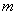falls on the very massive body with the mass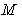, whose radius is equal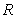(subsequently the bodyand the body). Let us assume that at the initial moment of time the distance between the bodies is very great and that is fulfilled the equation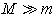. Let as to also consider that the density of the massive body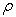. The rate of the fall of the bodyon the body surfacein this case can be found from the equation: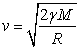(2.1)

where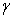is gravitational constant. If we switch over to substance density of massive body, then Eq. (2.1) can be rewritten as follows: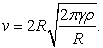(2.2)

Is obvious that kinetic energy, which possesses the falling body, it obtained from the gravitational field of the body. This kinetic energy of the falling body with its drop on the surface of massive body to become thermal energy will be radiated into the surrounding space in the form of electromagnetic waves.

From the aforesaid it is possible to conclude that the final summary mass of two bodies will not be equal to the sum of the masses of bodies prior to the beginning of the drop: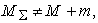i.e. there is a gravitational mass defect. The equation of the honey of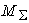and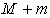can be found, knowing that kinetic energy, which possessed the bodywith the drop on the body. This energy can be calculated from the equation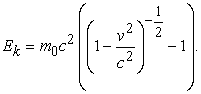During the record of this expression is taken into account the circumstance that with the fall of body in the gravitational field the acceleration of this body does not depend on its mass. Therefore

Eqs. (2.1) and (2.2) are accurate even for the relativistic speeds. It is now not difficult to calculate gravitational mass defect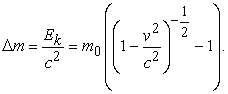(2.3)

This effect comprises with the drop on the earth's surface ~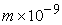.

From Eq. (31.3) is evident that the addition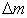can be both less and it is more than. If<, then with the fall of body summary mass increases. But if=, then an increase in the summary mass ceases, and entire mass of the falling body is converted into the thermal radiation. In this case massive body is converted into the ideal anvil, which converts entire mass of the falling body into the energy of electromagnetic radiation.

As can easily be seen Eq. (2.3), the rate of the fall of the body(let us name this speed of critical) to the body surfacewill be determined by the equation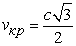(2.4)

i.e. it is considerably less than the speed of light.

If the density of massive body is known, then, using Eqs. (2.2) and (2.4), it is not difficult to find a critical radius of this body: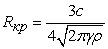By this concept we will understand the value of the radius, with reaching of which further increase in the mass of the bodydue to the fall on it of another body becomes impossible.

Can occur the situation for the space objects examined, for example for the neutron stars. It is known that the neutron stars (pulsars), have very high density . So pulsar with a mass ~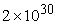kg (mass of the sun) would have a radius ~10 km. Its density in this case would compose ~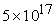kg/m3. With this density a critical radius would comprise near 15 km, and mass would compose ~ 3.4 of masses of the sun. This means that with reaching of such sizes and this mass the neutron star no longer can increase neither its sizes nor its mass, since any falling to it objects will be completely converted into the radiant energy.

According to preliminary calculations in our galaxy is counted about 300 thousand neutron stars . What to happen, if neutron star does encounter the same neutron star as it itself? It is obvious that the complete annihilation of neutron substance and its transformation into the energy will occur. Taking neutron star with a critical radius ~ 15 km and with the mass ~ 3.4 of masses of the sun, we obtain the value of energy 5 • 1047 J. This value of energy is very close to that energy, which characterizes explosion in the nucleus of galaxy NGC 3034 . During this explosion from the nucleus of galaxy was rejected a huge quantity of material throughout its mass equal 5,9 • 107 of the masses of the sun. This of phenomenon does not find its thus far explanation, since are not known those energy sources, which can lead to so immense an explosion. The process of the collision of neutron stars examined can be precisely such source.

In its essence this explosion this is the explosion of the nuclear charge of very large power. The isolation of such significant quantities of energy will be accompanied by warming-up and transformation into the plasma of large quantities of surrounding material. This in turn will lead to the appearance of the same electrical pour on as with the explosion of nuclear bomb, only much more significant. The presence of such pour on in the surrounding space they must lead to the appearance of specific polarization effects. To them can be attributed polarization in the electric fields of atoms and molecules and the appearance of the electric dipoles, which will lead to the polarization of the electromagnetic waves of those extending in the plasma.

### 3. Conclusion

In the article is examined the new physical phenomenon, which can be named gravitational mass defect. This phenomenon leads to the fact that the summary mass of bodies before and after the collisions differ. Indicated we will call mass defect gravitational mass defect and it can serve as a reason for explosions in the nuclei of galaxies.

### References

  J. P. Pskov. New andsupernovae, Moscow, 1985.In article  D. Yu. Tsvetkov. Supernovae, SAI, Moscow, 2001.In article  S. B. Popov. How toexplodegrown thingiants?, SAI, Moscow, 2011.In article  T. A. Agekyan. Stars, galaxy, metagalaxy. Publ. Science, 1981.In article  F. F.Mende. Problemsof modern physicsand their solutions, PALMARIUM Academic Publishing, 2012.In article